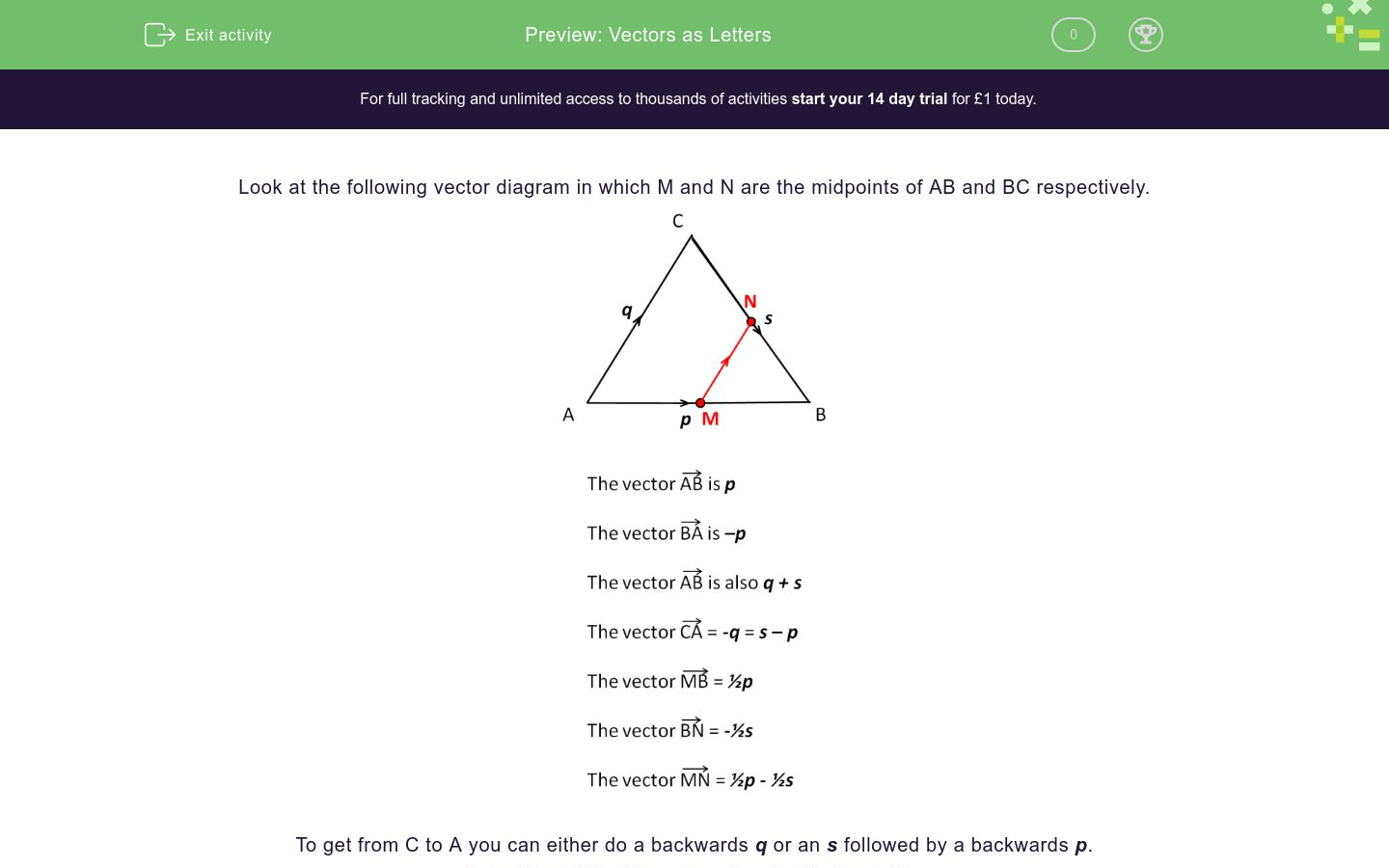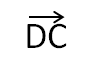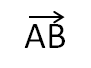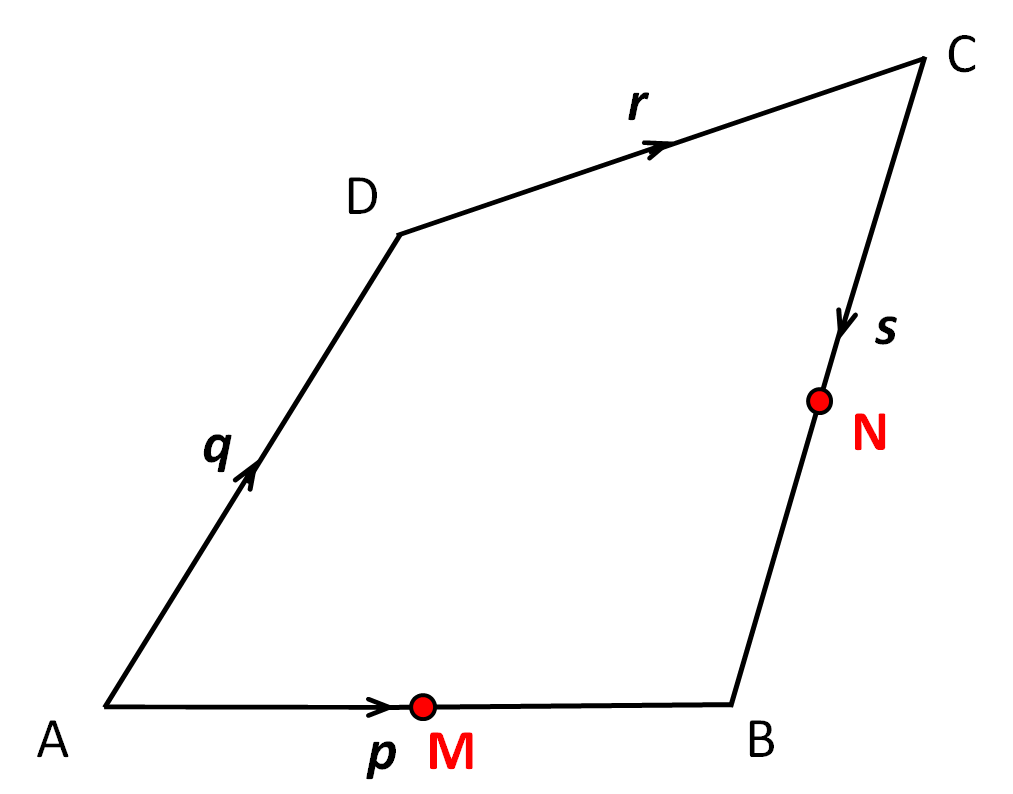# Vectors as Letters

In this worksheet, students must use letter notation to answer questions on vectors.Key stage:  KS 4

Curriculum topic:  Geometry and Measures

Difficulty level:### QUESTION 1 of 10

Look at the following vector diagram in which M and N are the midpoints of AB and BC respectively.To get from C to A you can either do a backwards q or an s followed by a backwards p.

To get from M to N you can also do -½p + q + ½s.

ABCD is a parallelogram.

The diagonals intersect at O.Select an expression, in terms of r and s, for the vector-s

2s

r - 2s

r - s

ABCD is a parallelogram.

The diagonals intersect at O.Select an expression, in terms of r and s, for the vector-s

2s

s - r

r - s

ABCD is a parallelogram.

The diagonals intersect at O.Select an expression, in terms of r and s, for the vector-s

2s

r - 2s

s - r

ABCD is a parallelogram.

The diagonals intersect at O.Select an expression, in terms of r and s, for the vector-s

2s

s - r

-r - s

ABCD is a quadrilateral.

M and N are the midpoints of AB and BC respectively.Select an expression, in terms of p, q, r and s, for the vector-s

½s

2s

½s

ABCD is a quadrilateral.

M and N are the midpoints of AB and BC respectively.Select an expression, in terms of p, q, r and s, for the vector-p

½p

-½p

½p

ABCD is a quadrilateral.

M and N are the midpoints of AB and BC respectively.Select an expression, in terms of p, q, r and s, for the vector½p - ½s

½p + ½s

-½p + s

½ps

ABCD is a quadrilateral.

L, M and N are the midpoints of AD, AB and BC respectively.Select an expression, in terms of r, s, t and u, for the vector½t - ½s

½s - ½t

-½t + s

½s + ½t

ABCD is a quadrilateral.

L, M and N are the midpoints of AD, AB and BC respectively.Select an expression, in terms of r, s, tand u, for the vector½s - ½r

½s - ½t

-½r + s

-r + s

ABCD is a quadrilateral.

L, M and N are the midpoints of AD, AB and BC respectively.Select all the expressions, in terms of r, s, tand u, for the vector½t - u - ½r

½s - u - ½t

½t - u - ½s

-½t - s + ½r

• Question 1

ABCD is a parallelogram.

The diagonals intersect at O.Select an expression, in terms of r and s, for the vector2s
EDDIE SAYS
Two lots of s.
• Question 2

ABCD is a parallelogram.

The diagonals intersect at O.Select an expression, in terms of r and s, for the vectors - r
EDDIE SAYS
Go from D to O and then from O to C, which is -r and then s.
• Question 3

ABCD is a parallelogram.

The diagonals intersect at O.Select an expression, in terms of r and s, for the vectors - r
EDDIE SAYS
This is the same vector as D to C.
• Question 4

ABCD is a parallelogram.

The diagonals intersect at O.Select an expression, in terms of r and s, for the vector-r - s
EDDIE SAYS
Go from C to O and then from O to B, which is -s and then -r.
• Question 5

ABCD is a quadrilateral.

M and N are the midpoints of AB and BC respectively.Select an expression, in terms of p, q, r and s, for the vector½s
EDDIE SAYS
This is half of s.
• Question 6

ABCD is a quadrilateral.

M and N are the midpoints of AB and BC respectively.Select an expression, in terms of p, q, r and s, for the vector-½p
EDDIE SAYS
This is half of p but backwards.
• Question 7

ABCD is a quadrilateral.

M and N are the midpoints of AB and BC respectively.Select an expression, in terms of p, q, r and s, for the vector½p - ½s
EDDIE SAYS
This is the same as M to B and then B to N, which is half of p and then half of -s.
• Question 8

ABCD is a quadrilateral.

L, M and N are the midpoints of AD, AB and BC respectively.Select an expression, in terms of r, s, t and u, for the vector½s + ½t
EDDIE SAYS
This is the same as M to B and then B to N, which is half of s and then half of t.
• Question 9

ABCD is a quadrilateral.

L, M and N are the midpoints of AD, AB and BC respectively.Select an expression, in terms of r, s, tand u, for the vector½s - ½r
EDDIE SAYS
This is the same as L to A and then A to M, which is half of -r and then half of s.
• Question 10

ABCD is a quadrilateral.

L, M and N are the midpoints of AD, AB and BC respectively.Select all the expressions, in terms of r, s, tand u, for the vector½t - u - ½r
-½t - s + ½r
EDDIE SAYS
There are two routes.
N to C then C to D then D to L, which is ½t - u - ½r
N to B then B to A then A to L, which is -½t - s + ½r
---- OR ----

Sign up for a £1 trial so you can track and measure your child's progress on this activity.

### What is EdPlace?

We're your National Curriculum aligned online education content provider helping each child succeed in English, maths and science from year 1 to GCSE. With an EdPlace account you’ll be able to track and measure progress, helping each child achieve their best. We build confidence and attainment by personalising each child’s learning at a level that suits them.

Start your £1 trial